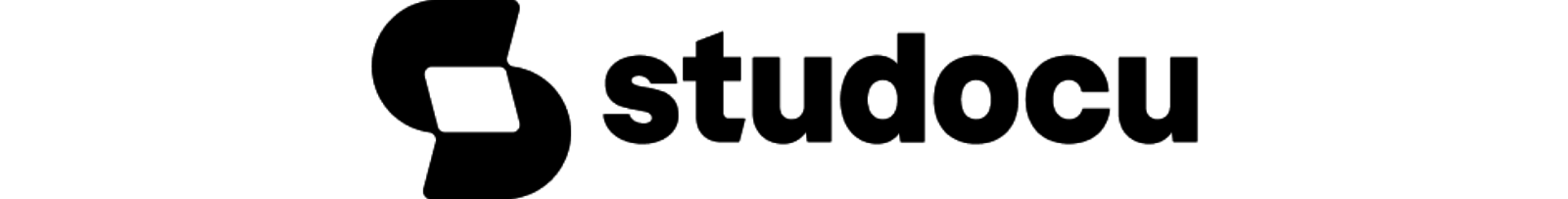Rating : ⭐⭐⭐⭐⭐
Price : \$10.99
Language:EN
Pages: 4

# The annual holding cost for each airplane the cost

lOMoARcPSD|24389855Using the continuous review system, what is the economic order quantity (EOQ. that Jon should use as the order quantity for this item? Select the closest number.

EXPLANATION

Gather data.

D = Annual Demand
D = Demand per week × # of weeks operating in a year D = 50 × 52
D = 2,600

S = Order Cost (Cost per order is already given) S = \$30

2 × 𝐷 × 𝑆
𝐸𝑂𝑄 = �
𝐻

2 × 2600 × 30
𝐸𝑂𝑄 = 10
𝐸𝑂𝑄 = � 156000

10

lOMoARcPSD|24389855

10,407 airplanes

207 airplanes

Now, calculate the Reorder Point.
Reorder Point = D × T + Safety Stock
Reorder Point = (50 × 4) + 7
Reorder Point = 200 + 7
Reorder Point = 207

Answer: Option 4 - 207 airplanes

How It Works